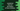# JavaScript program to find out the largest of three numbers## Introduction :

We can use different ways to find the largest among the three numbers in JavaScript. We can either use extra variables to find the largest or we can directly find without using any extra variables. In this blog post, I will show you different ways to find out the largest of three numbers in JavaScript. Try to go through the programs and drop one comment below if you have any queries.

## Example: Using extra variables :

The simplest way to find the largest of three variables is by using extra variables. Let’s take a look at the below example :

``````let firstNo = 1110;
let secondNo = 9120;
let thirdNo = 344;

let largerNo = firstNo > secondNo ? firstNo : secondNo;
let largestNo = largerNo > thirdNo ? largerNo : thirdNo;

console.log(`Largest number \${largestNo}`);
``````

Here, we are using two extra variables: largerNo and largestNo. The largerNo variable stores larger number among firstNo and secondNo. And, the largestNo variable stores the larger number among largerNo and largestNo i.e. it will contain the largest number among all.

## Example 2: Using if-else :

We can find out the largest of three numbers using multiple if-else conditions like below :

``````let firstNo = 1110;
let secondNo = 120;
let thirdNo = 3044;

if (firstNo > secondNo) {
if (firstNo > thirdNo) {
console.log(`\${firstNo} is the largest number`);
} else {
console.log(`\${thirdNo} is the largest number`);
}
} else {
if (secondNo > thirdNo) {
console.log(`\${secondNo} is the largest number`);
} else {
console.log(`\${thirdNo} is the largest number`);
}
}
``````

If the firstNo is greater than secondNo, check if firstNo is greater than thirdNo or not. Else, i.e. if firstNo is less than thirdNo, check if secondNo is greater than thirdNo or not. That’s it.

The main advantage of this program is that we are not using any extra variables to find out the largest.

## Using Math.max() :

JavaScript Math.max() function is used to find out the larger value of two numbers. We can also use this method to find out the largest among the three numbers.

The below JavaScript program uses Math.max() to find the largest among three numbers firstNo, secondNo and thirdNo. The inner Math.max function will find the larger number between firstNo and secondNo. The outer Math.max function will find the larger number between this result number and the thirdNo. So, it will give us the largest of these three numbers.

``````let firstNo = 10;
let secondNo = 20;
let thirdNo = 344;

let largestNo = Math.max(Math.max(firstNo,secondNo),thirdNo)

console.log(`Largest number \${largestNo}`);
``````